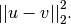# scipy.spatial.distance.sqeuclidean¶

scipy.spatial.distance.sqeuclidean(u, v)[source]

Computes the squared Euclidean distance between two 1-D arrays.

The squared Euclidean distance between u and v is defined asParameters : u : (N,) array_like Input array. v : (N,) array_like Input array. sqeuclidean : double The squared Euclidean distance between vectors u and v.

#### Previous topic

scipy.spatial.distance.sokalsneath

#### Next topic

scipy.spatial.distance.wminkowski